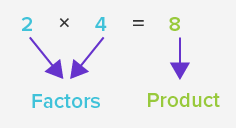Search

# Don’t Forget Your Math Basics!

Updated: Jan 10

By: Rita Barakat

Edited: by Henry Fleischacker

Helping your child with math homework can seem frustrating at times for various reasons: the method your child is being taught in school is different from what you were taught, your child doesn’t seem to be understanding your explanation of the concepts, etc.

In my time as a teacher and tutor, I’ve noticed the most significant thing hindering students is, for whatever reason, a neglect of the basics.

Here are the foundational math concepts to review with your kids to make math homework a breeze!

1. Which Pairs of Numbers Add Up to 10?Knowing these makes mental math and adding larger numbers much easier!

Take 176 + 204. Knowing that 6 + 4 = 10, you can move the 4 from the 204 to the 176 to get 180 + 200 = 380. That is much easier to work with!

1. How Do You Skip Count?Skip counting is an excellent way to practice both addition and multiplication. If your child can skip-count each number group in under 10 seconds, fantastic! If you want to challenge them further, try to get them to skip count each group in under 6 seconds.

1. What Is A Factor?

A factor is a number that can be multiplied by another number to get a product.

For example: 4 is a factor of 12 because 4 x 3 = 12Practicing factors is a great way to practice multiplication facts as well as introduce division!

1. What Is A Multiple?

A multiple is a number that can be divided by the original number evenly.

For example: 18 is a multiple of 6 because 18 divided by 6 is 3 with no remainder.This picture shows the multiples of 8 up to 48.

With these foundational math skills mastered, your child is set up for success in the areas of PEMDAS, integers, fractions, and decimals that build up into equations, algebra, geometry, and calculus.